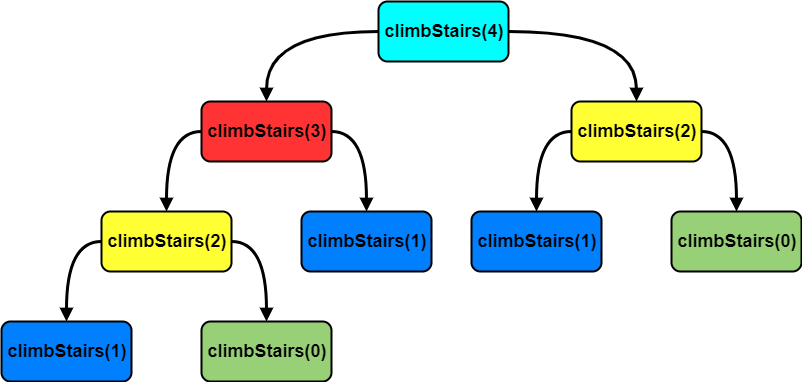# Coding Patterns: Staircase (DP)

In Coding Patterns series, we will try to recognize common patterns underlying behind each algorithm question, using real examples from Leetcode.

Previous posts were about Sliding Window, Two Pointers, Fast & Slow Pointers, Merge Intervals, Cyclic Sort, In-place Reversal of a Linked List, Breadth First Search (BFS), Depth First Search (DFS), Two Heaps, Subsets, Modified Binary Search, Top K Numbers, K-way Merge, 0/1 Knapsack, Topological Sort and Bitwise XOR patterns and today, we will introduce Staircase pattern which is very useful to solve Dynamic Programming problems involving minimum/maximum steps, jumps, stairs, fibonacci numbers etc. to reach a target.

If you need to refresh your knowledge of Dynamic Programming, you may want to check the Fibonacci Number Problem before diving into more advanced problems.

## Problem: Climbing Stairs

LeetCode 70 - Climbing Stairs [easy]

You are climbing a stair case. It takes n steps to reach to the top. Each time you can either climb 1 or 2 steps. In how many distinct ways can you climb to the top?

Note:

Given n will be a positive integer.

Example 1:

``````Input: 2
Output: 2
Explanation: There are two ways to climb to the top.
--> 1 step + 1 step
--> 2 steps
``````

Example 2:

``````Input: 3
Output: 3
Explanation: There are three ways to climb to the top.
--> 1 step + 1 step + 1 step
--> 1 step + 2 steps
--> 2 steps + 1 step
``````

### Brute Force Solution

At every step, we have two options: either climb 1 step or 2 steps.

``````class Solution:
def climbStairs(self, n: int) -> int:
# we don't take any steps, so there is only 1 way
if n == 0:
return 0
# we can take one step to reach the end, and this is the only way
if n == 1:
return 1
# we can take one step twice or take two steps to reach the end
if n == 2:
return 2

# if we take one step, we are left with "n - 1" steps
take1step = self.climbStairs(n - 1)
# if we take two steps, we are left with "n - 2" steps
take2steps = self.climbStairs(n - 2)

return take1step + take2steps
``````

Time Complexity: O(2N) because we are making 2 recursive calls in the same function.

Space Complexity: O(N) which is used to store the recursion stack.

But can we do better? To be able to understand this, lets visualize the recursion stack.We can clearly see from colors that there are lots of overlapping sub-problems that we don’t need to calculate them again and again.

### Top-down Dynamic Programming with Memoization

We can use an array to store already solved sub-problems.

``````class Solution:
def climbStairs(self, n: int) -> int:
dp = [0 for x in range(n+1)]
return self.climbStairs_recursive(dp, n)

def climbStairs_recursive(self, dp, n):
# we don't take any steps, so there is only 1 way
if n == 0:
return 0
# we can take one step to reach the end, and this is the only way
if n == 1:
return 1
# we can take one step twice or take two steps to reach the end
if n == 2:
return 2

if dp[n] == 0:
# if we take one step, we are left with "n - 1" steps
take1step = self.climbStairs_recursive(dp, n - 1)
# if we take two steps, we are left with "n - 2" steps
take2steps = self.climbStairs_recursive(dp, n - 2)

dp[n] = take1step + take2steps

return dp[n]
``````

Time Complexity: O(N) because memoization array `dp[n+1]` stores the results of all sub-problems. We can conclude that we will not have more than `n + 1` sub-problems.

Space Complexity: O(N) which is used to store the recursion stack.

### Bottom-up Dynamic Programming with Tabulation

Let’s try to populate our `dp[]` array in a bottom-up fashion. As we see from the recursion stack visualization, each `climbStairs(n)` call is the sum of the two previous calls.

We can use this fact while populating our `dp[]` array.

``````class Solution:
def climbStairs(self, n: int) -> int:
dp = [0 for x in range(n+1)]
dp = 1
dp = 1

for i in range(2, n+1):
dp[i] = dp[i-1] + dp[i-2]

return dp[n]
``````

Time Complexity: O(N)

Space Complexity: O(N) which is used to store the recursion stack.

### Memory Optimization

As we can see from the visualization of the recursive stack and other solutions, to be able to calculate the n, you need the value of last two combinations: n-1 and n-2.

These two values are enough and we don’t need to store all other past combinations.

``````class Solution:
def climbStairs(self, n: int) -> int:
if n <= 0:
return 0
if n == 1:
return 1
if n == 2:
return 2

first = 1  # how many step possibilities there are with 1 stairs
second = 2  # how many step possibilities there are with 2 stairs
third = 0

for _ in range(2, n):
third = first + second
first = second  # walk up first to second
second = third  # walk up second to third
return third
``````

OR more shortly;

``````class Solution:
def climbStairs(self, n: int) -> int:
a = b = 1
for _ in range(n):
a, b = b, a + b
return a
``````

Time Complexity: O(N)

Space Complexity: O(1)

## How to identify?

Staircase pattern is very useful to solve Dynamic Programming problems involving minimum/maximum steps, jumps, stairs, fibonacci numbers etc. to reach a target.

Tags:

Categories:

Updated: